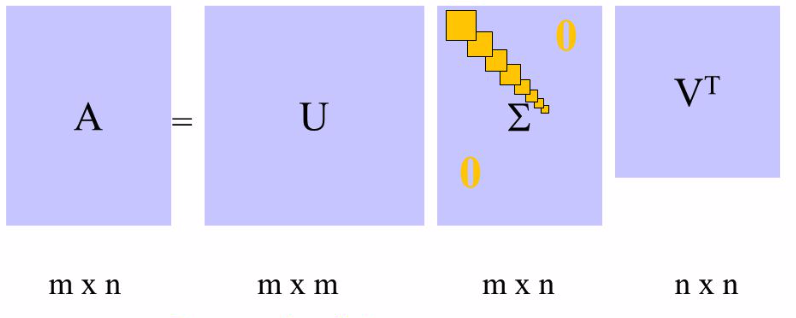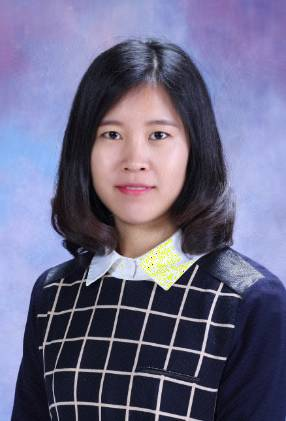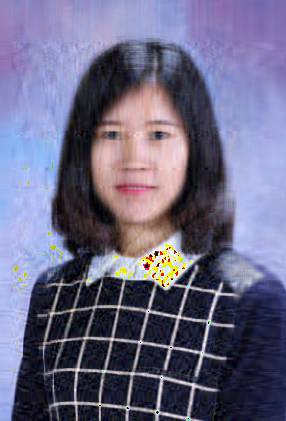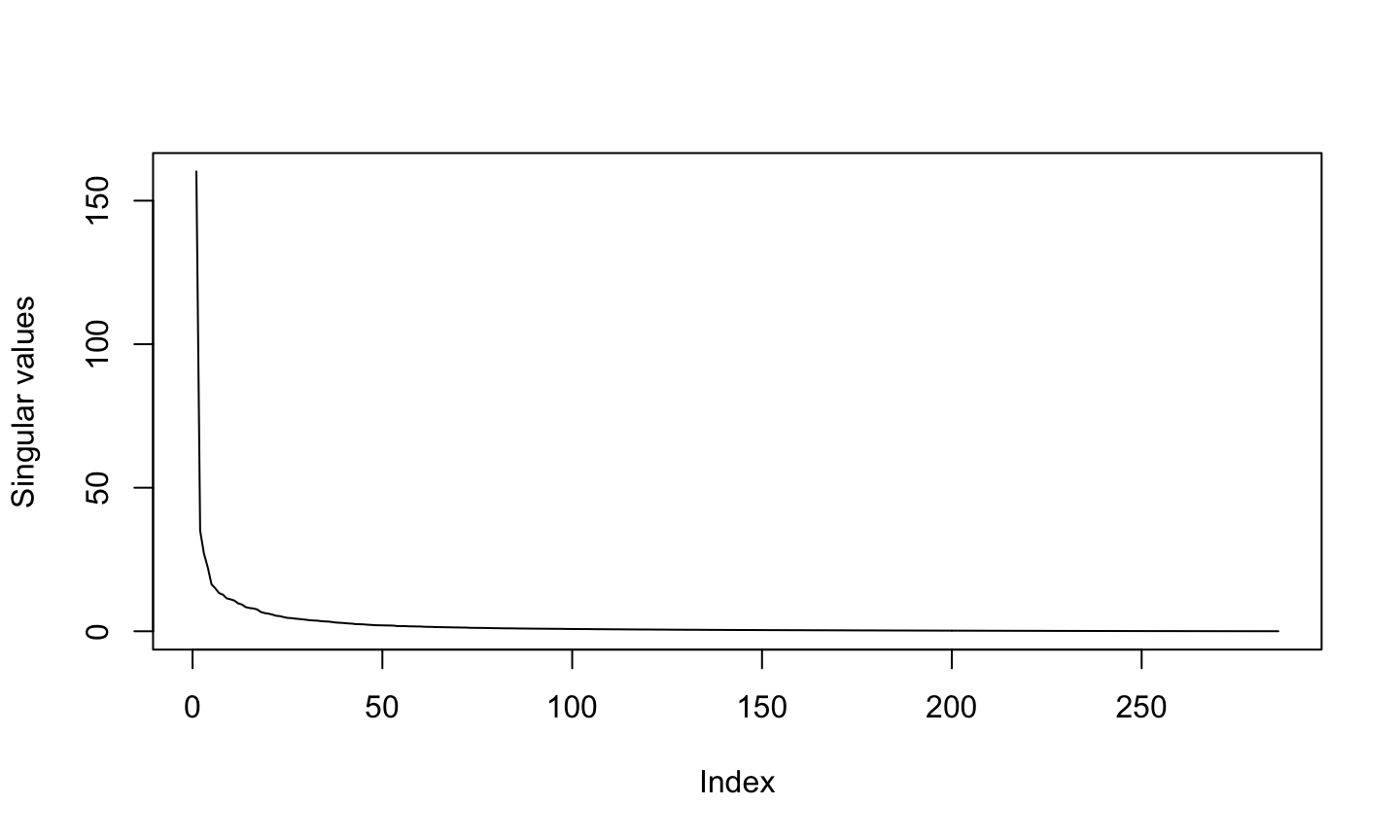School of Economics and Management
Beihang University
http://yanfei.site

## Applications of SVD

• In data compression, we start with a matrix A that contains perfect data, and we try to find a (lower-rank) approximation to the data that seeks to capture the principal elements of the data.
• we sacrifice only the less important parts that don’t degrade data quality too much, in order to gain compression.
• In noise filtering, we start with a matrix A that contains imperfect data, and we try to find a (lower-rank) approximation to the data that seeks to capture the principal elements of the data.
• we give up only the less important parts that are typically noise.
• So both these tasks are related, and rely on the SVD to find a suitable lower-ranked approximation to a matrix

## Matrix Approximation

• Recall that the SVD of a matrix $$A$$ in $$\mathcal{R}_{m\times n}$$ decomposes the matrix into $A = U\Sigma V^T = \left(\begin{array}{cc}U_1 & U_2\end{array}\right) \left(\begin{array}{cc} D & \\ & 0 \end{array}\right) \left(\begin{array}{cc}V_1^T & V_2^T\end{array}\right) = U_1^TDV_1^T$ where $$D = \text{diag}\{\alpha_1, \cdots, \alpha_r\} \in \mathcal{R}^{r\times r}$$ with $$\sigma_r > 0$$ for $$r = \text{rank}(A)$$.

• $$A$$ can also be expressed as a sum of outer products, with each sum being a rank 1 matrix of dimension $$m\times n$$ $A = \Sigma_{i = 1}^r \sigma_i u_u v_i^T = \alpha_1 u_1 v_1^T + \cdots + \alpha_r u_r v_r^T.$

• We can truncate this sum when we feel that the singular values are so small that they are not contributing much.

## SVD components## Matrix Approximation Error

• So if we truncate the sum after $$k$$ singular values, then we are approximating $$A$$ with $$A_k$$

$A_k = \Sigma_{i = 1}^{k<r} \sigma_i u_u v_i^T = \alpha_1 u_1 v_1^T + \cdots + \alpha_k u_k v_k^T.$

• The error in the approximation is $$\sigma_{k+1}$$.

• We have $$\text{rank}(A_k) = k < r = \text{rank}(A).$$

• By approximating $$A$$ with $$A_k$$, we have saved memory:
• $$A$$ requires us to store $$m\times n$$ numbers
• $$A_k$$ requires us to store ??? numbers?

– Significant savings for large matrices, and/or small $$k$$.

## Image Compression

• Suppose we have a grayscale image ($$128 \times 128$$ pixels).
• We can use a matrix to represent this image.
• If we have a colour image, it has three matrices, with the same size as the image. Each matrix represents a color value that comprises the RGB color scale.
• Each pixel is represented by an integer from 0-255.
• Next, we can decompose the matrix by SVD.
• By eliminating small singular values, we can approximate the matrix.
• Choose the value of $$k$$ for the low-rank approximation $$A_k$$.
• Plotting the singular values might help identify where there is a noticeable drop in significance

## Reconstructing approximated image

• Suppose we have chosen the value of $$k$$ = number of singular values we wish to retain. - We can generate a new image matrix by expanding $$A$$ using the SVD (first $$k$$ singular values only).
• If you want to use colour images, do it for R, G, B matrices separately and then reassemble.

## Data compression in R

Here are four images with rank 286, 200, 116 and 32. How many numbers to store for each of them?## Plot of singular values## Lab session

• Like in today’s lecture, take a high resolution image of yourself, and produce a sequence of low rank approximations.
• How much can you save?

## Noise reduction

• The SVD also has applications in digital signal processing
• The central idea is to let a matrix $$A$$ represent the noisy signal, compute the SVD, and then discard small singular values of $$A$$.
• It can be shown that the small singular values mainly represent the noise, and thus the rank $$k$$ matrix $$A_k$$ represents a filtered signal with less noise.

## Application to Signal Separation

• Suppose we have $$P$$ observed signals $$m_i(t)$$ which are linear combinations of r source signals $$s_i(t)$$, corrupted by noise signals $$n_i(t)$$. We have a time series of data from $$T$$ time periods
• This can be written as: $m_i(t) = \alpha_{i1}s_1(t) + \cdots + a_{ir}s_r(t) + n_i(t),$ where $$t = 1, \cdots, T$$ and $$i = 1, \cdots, P$$.
• Write the equivalent matrix representation.

## SVD of signal matrix $$M$$

• We can decompose $$M$$ using SVD: $$M = U\Sigma V^T$$.
• If the signal compared to the noise is sufficiently strong, we are likely to find clear distinction between the singular values due to the signal and those due to noise $U = (U_s, U_n),~\Sigma = \left(\begin{array}{cc} \Sigma_s & \\ & \Sigma_n \end{array}\right),~V^T = \left(\begin{array}{c} V_s \\ V_n \end{array}\right)$

## Results of noise reduction

library(bootSVD)
set.seed(1)
Y <- simEEG(n = 100, centered = TRUE, propVarNoise = 0.3, wide = TRUE)
svdY <- svd(Y)
par(mfrow = c(2, 2))
for (j in c(3, 20, 50, 100)) {
a = svdY$u[, 1:j] %*% diag(svdY$d[1:j]) %*% t(svdY\$v[, 1:j])
plot(a[1, ], type = "l", xlab = "", ylab = "signal", main = paste("r = ",
j, sep = ""))
}